## Kaggle 入门并实战房价预测

今天看了个新闻，说是中国社会科学院城市发展与环境研究所及社会科学文献出版社共同发布《房地产蓝皮书：中国房地产发展报告No.16(2019)》指出房价上涨7.6%，看得我都坐不住了，这房价上涨什么时候是个头啊。为了让自己以后租得起房，我还是好好努力吧。于是我打开了Kaggle，准备上手第一道题，正巧发现有个房价预测，可能这是命运的安排吧......

## 一、下载数据

进入到 kaggle 后要先登录，需要注意的是，注册的时候有一个验证，要FQ才会显示验证信息。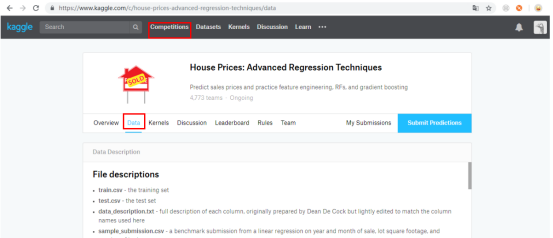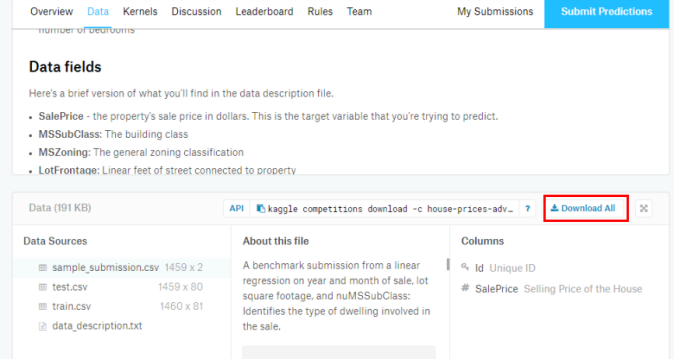下载好数据之后，大致看一下数据的情况，在对应题目的页面也有关于数据属性的一些解释，看一下对应数据代表什么。

## 二、数据预处理

### 提取 y_train 并做相应处理

先导入需要用到的包，通过 pandas 的 read_csv(filename, index_col=0) 分别将测试集和训练集导入。完了之后，我们把训练集里的“SalePrice”取出来，查看它的分布情况并作一下处理。

```y_train = train_data.pop('SalePrice')
y_train.hist()```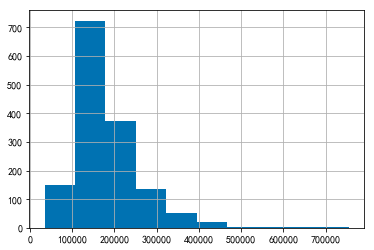由此可见数据并不平滑，因此需要将其正态化，正态化可以使数据变得平滑，目的是稳定方差，直线化，使数据分布正态或者接近正态。正态化可以用 numpy 的 log1p() 处理。log1p(y_train) 可以理解为 log 1 plus，即 log（y_train + 1）。正态化之前，先看一下如果正态化之后的价格分布。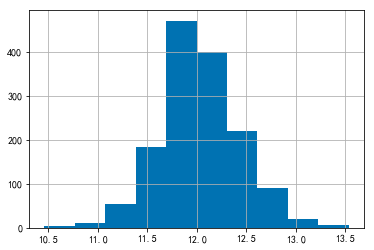这样的分布就很好了，因此我们通过 numpy 的 log1p() 将 y_train 正态化。

`y_train = np.log1p(y_train)`

### 将去掉 SalePrice 的训练集和测试集合并

为了将两个数据集一起处理，减少重复的步骤，将两个数据集合并再处理。使用 pandas 的 concat() 将训练集和测试集合并起来并看一下合并后的数据的行数和列数，以确保正确合并。

```data = pd.concat((train_data, test_data), axis=0)
data.shape```

### 特征处理

数据集中有几个跟年份有关的属性，分别是：

• YrSold: 售出房子的年份；
• YearBuilt：房子建成的年份；
• GarageYrBlt：车库建成的年份

算出跟售出房子的时间差，并新生成单独的列，然后删除这些年份

```data.eval('Built2Sold = YrSold-YearBuilt', inplace=True)
data.eval('GarageBlt = YrSold-GarageYrBlt', inplace=True)
data.drop(['YrSold', 'YearBuilt', 'YearRemodAdd', 'GarageYrBlt'], axis=1, inplace=True)```

接下来进行变量转换，由于有一些列是类别型的，但由于pandas的特性，数字符号会被默认成数字。比如下面三列，是以数字来表示等级的，但被认为是数字，这样就会使得预测受到影响。

• OverallQual: Rates the overall material and finish of the house
• OverallCond: Rates the overall condition of the house
• MSSubClass: The building class

这三个相当于是等级和类别，只不过是用数字来当等级的高低而已。因此我们要把这些转换成 string

```data['OverallQual'] = data['OverallQual'].astype(str)
data['OverallCond'] = data['OverallCond'].astype(str)
data['MSSubClass'] = data['MSSubClass'].astype(str)```

### 把category的变量转变成numerical

我们可以用One-Hot的方法来表达category。pandas自带的get_dummies方法，可以一键做到One-Hot。

这里按我的理解解释一下One-Hot：比如说有一组自拟的数据 data，其中 data['学历要求']有'大专', '本科', '硕士', '不限'。但data['学历要求']=='本科'，则他可以用字典表示成这样{'大专': 0, '本科':1, '硕士':0, '不限':0}，用向量表示为[0, 1, 0, 0]

`dummied_data = pd.get_dummies(data)`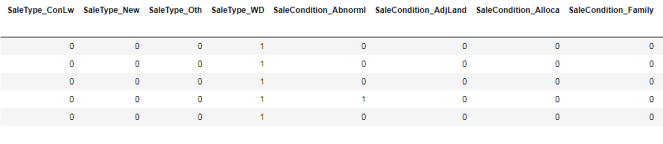### 处理numerical变量

category变量处理好了之后，就该轮到numerical变量了。查看一下缺失值情况。

`dummied_data.isnull().sum().sort_values(ascending=False).head()`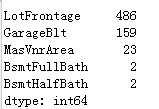上面的数据显示的是每列对应的缺失值情况，对于缺失值，需要进行填充，可以使用平均值进行填充。

```mean_cols = dummied_data.mean()
dummied_data = dummied_data.fillna(mean_cols)```

### 标准差标准化

缺失值处理完毕，由于有一些数据的值比较大，特别是比起 one-hot 后的数值 0 和 1，那些几千的值就相对比较大了。因此对数值型变量进行标准化。

```numerical_cols = data.columns[data.dtypes != 'object']  # 数据为数值型的列名
num_cols_mean = dummied_data.loc[:, numerical_cols].mean()
num_cols_std = dummied_data.loc[:, numerical_cols].std()
dummied_data.loc[:, numerical_cols] = (dummied_data.loc[:, numerical_cols] - num_cols_mean) / num_cols_std```

到这里，数据处理算是完毕了。虽然这样处理还不够完善，后面如果技术再精进一点可能会重新弄一下。接下来需要将数据集分开，分成训练集合测试集。

```X_train = dummied_data.loc[train_data.index].values
X_test = dummied_data.loc[test_data.index].values```

## 三、建模预测

由于这是一个回归问题，我用 sklearn.selection 的 cross_val_score 试了岭回归（Ridge Regression）、BaggingRegressor 以及 XGBoost。且不说集成算法比单个回归模型好，XGBoost  不愧是 Kaggle 神器，效果比 BaggingRegressor 还要好很多。安装 XGBoost  的过程就不说了，安装好之后导入包就行了，但是我们还要调一下参。

```params = [6,7,8]
scores = []
for param in params:
model = XGBRegressor(max_depth=param)
score = np.sqrt(-cross_val_score(model, X_train, y_train, cv=10, scoring='neg_mean_squared_error'))
scores.append(np.mean(score))
plt.plot(params, scores)```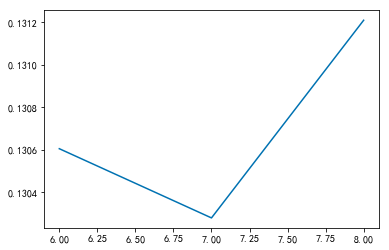可见当 max_depth = 7 的时候，错误率最低。接下来就是建模训练预测了。

```xgbr = XGBRegressor(max_depth=7)
xgbr.fit(X_train, y_train)
y_prediction = np.expm1(xgbr.predict(X_test))```

得到结果之后，将结果保存为 .csv 文件，因为 kaggle 的提交要求是 csv 文件，可以到 kaggle 看一下提交要求，前面下载的文件里面也有一份提交样式，照它的格式保存文件就好了。

```submitted_data = pd.DataFrame(data= {'Id' : test_data.index, 'SalePrice': y_prediction})
submitted_data.to_csv('./input/submission.csv', index=False)　　# 将预测结果保存到文件```

## 四、提交结果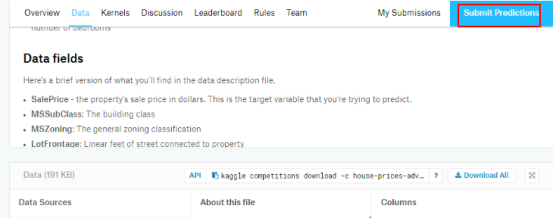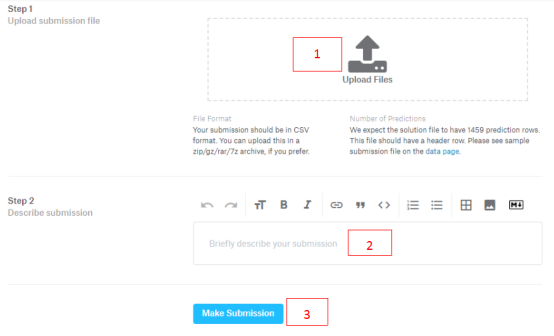提交的时候也要FQ，才会上传文件。到这里就结束了。

想要第一时间获取更多有意思的推文，可关注公众号： Max的日常操作